Next: Exercises Up: Electrostatics in Dielectric Media Previous: Clausius-Mossotti Relation

# Dielectric Liquids in Electrostatic Fields

Consider the behavior of an uncharged dielectric liquid placed in an electrostatic field. If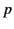is the pressure within the liquid when in equilibrium with the electrostatic force density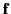then force balance requires that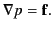(599)

It follows from Equation (585) that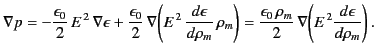(600)

We can integrate this equation to give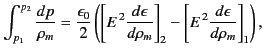(601)

where 1 and 2 refer to two general points in the liquid. Here, it is assumed that the liquid possesses an equation of state, so that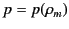. If the liquid is essentially incompressible (i.e.,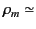constant) then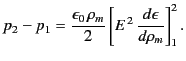(602)

Finally, if the liquid obeys the Clausius-Mossotti relation then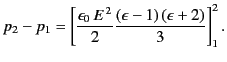(603)

According to Equations (554) and (604), if a sphere of dielectric liquid is placed in a uniform electric field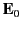then the pressure inside the liquid takes the constant value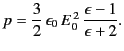(604)

It is clear that the electrostatic forces acting on the dielectric are all concentrated at the edge of the sphere, and are directed radially inwards: that is, the dielectric is compressed by the external electric field. This is a somewhat surprising result because the electrostatic forces acting on a rigid conducting sphere are concentrated at the edge of the sphere, but are directed radially outwards. We might expect these two cases to give the same result in the limit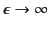. The reason that this does not occur is because a dielectric liquid is slightly compressible, and is, therefore, subject to an electrostriction force. There is no electrostriction force for the case of a completely rigid body. In fact, the force density inside a rigid dielectric (for which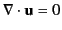) is given by Equation (585), with the third term (the electrostriction term) missing. It is easily demonstrated that the force exerted by an electric field on a rigid dielectric is directed outwards, and approaches that exerted on a rigid conductor in the limit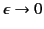.

As is well known, if a pair of charged (parallel plane) capacitor plates are dipped into a dielectric liquid then the liquid is drawn up between the plates to some extent. Let us examine this effect. We can, without loss of generality, assume that the transition from dielectric to vacuum takes place in a continuous manner. Consider the electrostatic pressure difference between a pointlying just above the surface of the liquid in between the plates, and a point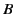lying just above the surface of the liquid well away from the capacitor (where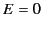). The pressure difference is given by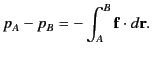(605)

Note, however, that the Clausius-Mossotti relation yields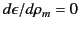at bothand, because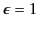in a vacuum [see Equation (599)]. Thus, it is clear from Equation (585) that the electrostriction term makes no contribution to the line integral (606). It follows that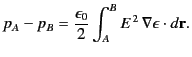(606)

The only contribution to this integral comes from the vacuum/dielectric interface in the vicinity of point(because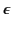is constant inside the liquid, andin the vicinity of point). Suppose that the electric field at pointhas normal and tangential (to the surface) components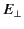and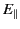, respectively. Making use of the boundary conditions that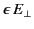andare constant across a vacuum/dielectric interface, we obtain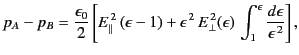(607)

giving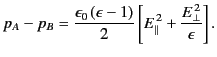(608)

This electrostatic pressure difference can be equated to the hydrostatic pressure difference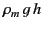to determine the height,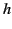, that the liquid rises between the plates. At first sight, the above analysis appears to suggest that the dielectric liquid is drawn upward by a surface force acting on the vacuum/dielectric interface in the region between the plates. In fact, this is far from being the case. A brief examination of Equation (604) shows that this surface force is actually directed downwards. According to Equation (585), the force which causes the liquid to rise between the plates is a volume force that develops in the region of non-uniform electric field at the base of the capacitor, where the field splays out between the plates. Thus, although we can determine the height to which the fluid rises between the plates without reference to the electrostriction force, it is, somewhat paradoxically, this force that is actually responsible for supporting the liquid against gravity.

Let us consider another paradox concerning the electrostatic forces exerted in a dielectric medium. Suppose that we have two charges embedded in a uniform dielectric of dielectric constant. The electric field generated by each charge is the same as that in a vacuum, except that it is reduced by a factor. We, therefore, expect the force exerted by one charge on the other to be the same as that in a vacuum, except that it is also reduced by a factor. Let us examine how this reduction in force comes about. Consider a simple example. Suppose that we take a parallel plate capacitor, and insert a block of solid dielectric between the plates. Suppose, further, that there is a small vacuum gap between the faces of the block and each of the capacitor plates. Let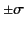be the surface charge densities on each of the capacitor plates, and let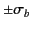be the bound charge densities that develop on the outer faces of the intervening dielectric block. The two layers of bound charge produce equal and opposite electric fields on each plate, and their effects therefore cancel each other. Thus, from the point of view of electrical interaction alone there would appear to be no change in the force exerted by one capacitor plate on the other when a dielectric slab is placed between them (assuming that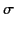remains constant during this process). That is, the force per unit area (which is attractive) remains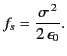(609)

However, in experiments in which a capacitor is submerged in a dielectric liquid the force per unit area exerted by one plate on another is observed to decrease to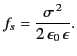(610)

This apparent paradox can be explained by taking into account the difference in liquid pressure in the field-filled space between the plates, and the field-free region outside the capacitor. This pressure difference is balanced by internal elastic forces in the case of the solid dielectric discussed earlier, but is transmitted to the plates in the case of the liquid. We can compute the pressure difference between a pointon the inside surface of one of the capacitor plates, and a pointon the outside surface of the same plate using Equation (607). If we neglect end effects then the electric field is normal to the plates in the region between the plates, and is zero everywhere else. Thus, the only contribution to the line integral (607) comes from the plate/dielectric interface in the vicinity of point. Using Equation (609), we find that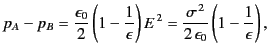(611)

where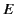is the normal field-strength between the plates in the absence of dielectric. The sum of this pressure force and the purely electrical force (610) yields a net attractive force per unit area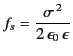(612)

acting between the plates. Thus, any decrease in the forces exerted by charges on one another when they are immersed or embedded in a dielectric medium can only be understood in terms of mechanical forces transmitted between these charges by the medium itself.Next: Exercises Up: Electrostatics in Dielectric Media Previous: Clausius-Mossotti Relation
Richard Fitzpatrick 2014-06-27